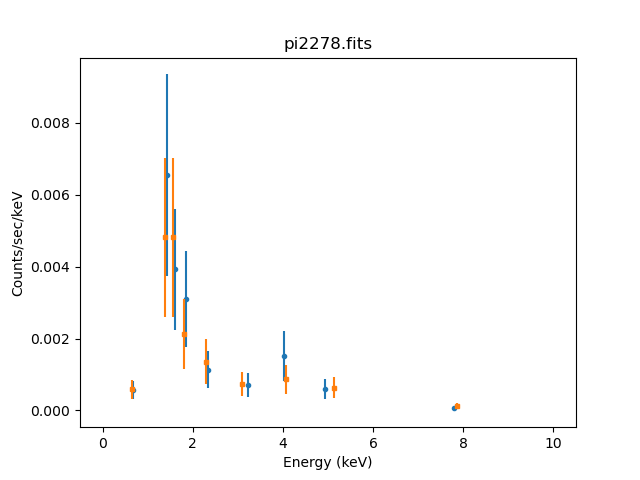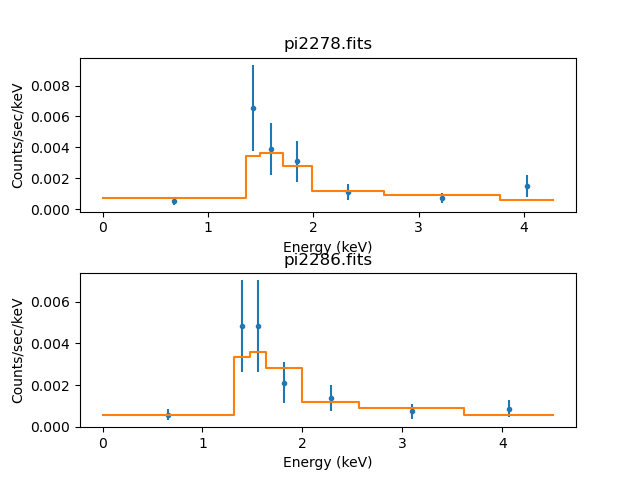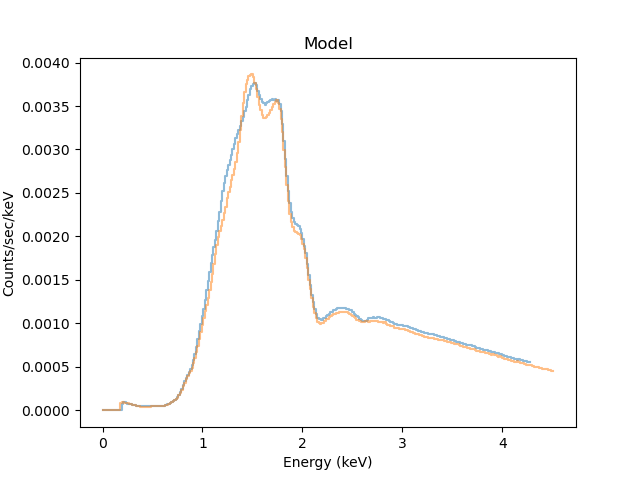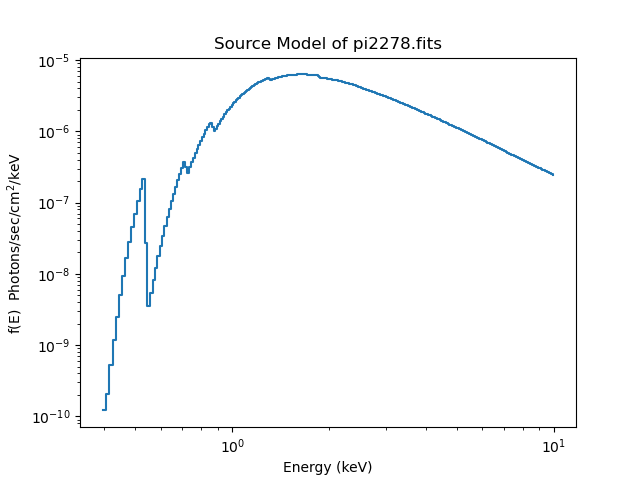# Simultaneously Fitting Two Data Sets## Overview

#### Synopsis:

The Sherpa syntax easily allows the modeling of multiple, independent data sets. In this example, we have a source observed on two different occasions. If we assume that the source is constant, then the only change will be in the instrument response. We account for this by defining each spectrum independently and fitting with the same model. This allows us to increase the significance of our fit, without making assumptions inherent in the process of averaging spectra and responses.

This thread may also be used to fit source and background data which are independently grouped. Note that the "standard method" of doing this is described in the Simultaneously Fitting Source and Background Spectra thread.

While the sample data files used in this thread are available as sherpa.tar.gz, note also that they are generated with the Extract ACIS Spectra and Response Files for Pointlike Sources thread.

Last Update: 5 Dec 2022 - reviewed for CIAO 4.15, screen output updated; typos fixed.

## Statistical Issues: Background Subtraction

A typical dataset may contain multiple spectra, one of which contains contributions from the source and the background, and one or more which contain background counts alone. (The background itself may contain contributions from the cosmic X-ray background, the particle background, and so on, but we ignore this complication.)

The proper way to treat background data is to model them. However, many X-ray astronomers subtract background data from the raw data.

Why should one not subtract background?

• It reduces the amount of statistical information in the analysis—the final fit parameter values will be a less accurate estimate of the true values.
• The background-subtracted data are not Poisson-distributed; one cannot fit them with the Poisson likelihood or the Cash statistic, even in the low-counts limit. For example, subtracting a background can give negative counts; this is definitely not Poissonian!
• Fluctuations, particularly in the vicinity of localized features, can adversely affect analysis.

Simultaneous fitting is identical to the basic method of fitting data, except for the specification of the data set ID. Most Sherpa commands have the option of specifying the ID of the data set on which to invoke the command. By default, this number is assumed to be 1; the ID is assigned when the data are loaded into Sherpa.

The spectral data from the two observations of the source are stored in the FITS files pi2278.fits and pi2286.fits. Data Set pi2278.fits is input with the load_pha() command and given ID "1":

```sherpa> load_pha(1, "pi2278.fits")
statistical errors were found in file 'pi2278.fits'
but not used; to use them, re-read with use_errors=True
```

The second data set is loaded similarly, but is given ID "2":

```sherpa> load_pha(2, "pi2286.fits")
statistical errors were found in file 'pi2286.fits'
but not used; to use them, re-read with use_errors=True
```

## Defining Instrument Responses

Sherpa will automatically read in any instrument response files that are defined in the header of the spectrum (keywords RESPFILE and ANCRFILE).

In this example, the response files were not defined, so we load them with load_arf() and load_rmf(). The data set ID of the corresponding PI file is given in each command:

```sherpa> load_arf(1, "arf2278.fits")
```

and

```sherpa> load_arf(2, "arf2286.fits")
```

With the responses loaded (so that Sherpa can convert between channel values and energy) we can display the data in physically-meaningful units (Figure 1):

```sherpa> plot_data(1)
sherpa> plot_data(2, marker='s', markersize=3, overplot=True)
```

### Figure 1: The two spectra### Figure 1: The two spectra

The blue points refer to the first dataset and the orange points to the second dataset. As we set the marker option for the second dataset the symbol was changed to a "s" (a square; see the matlpotlib marker documentation for the possible options).

## Defining Source Models

We want to model each of the spectra with an absorbed power-law, so a power-law component (powlaw1d, called pl1) and an absorbing component (xsphabs, called abs1) are defined. The same model expression is assigned to each data set:

```sherpa> set_source(1, xsphabs.abs1 * powlaw1d.pl1)
sherpa> set_source(2, abs1 * pl1)
```

Data above 5.5 keV is ignored in both data sets to cut out energies with no data. While this is not strictly necessary for this data, it does produce a nicer plot. Ignoring the higher energies may be especially useful for ACIS-S data, where the high-energy background is stronger.

The comma after the value indicates an open range, i.e. everything above "5.5" [keV] will be ignored:

```sherpa> ignore(5.5,)
dataset 1: 0:10.001 -> 0:4.2778 Energy (keV)
dataset 2: 0:10.001 -> 0:4.5114 Energy (keV)
```

We can use the Sherpa guess command to guess the initial parameter values and ranges for the power-law model component. To have Sherpa automatically query for the initial parameter values when a model is established, set paramprompt(True) (it is False by default).

```sherpa> guess(pl1)
sherpa> show_model()
Model: 1
apply_rmf(apply_arf((11619.081430486 * (xsphabs.abs1 * powlaw1d.pl1))))
Param        Type          Value          Min          Max      Units
-----        ----          -----          ---          ---      -----
abs1.nH      thawed            1            0       100000 10^22 atoms / cm^2
pl1.gamma    thawed            1          -10           10
pl1.ref      frozen            1 -3.40282e+38  3.40282e+38
pl1.ampl     thawed   6.4442e-06   6.4442e-09    0.0064442

Model: 2
apply_rmf(apply_arf((11619.081410727 * (xsphabs.abs1 * powlaw1d.pl1))))
Param        Type          Value          Min          Max      Units
-----        ----          -----          ---          ---      -----
abs1.nH      thawed            1            0       100000 10^22 atoms / cm^2
pl1.gamma    thawed            1          -10           10
pl1.ref      frozen            1 -3.40282e+38  3.40282e+38
pl1.ampl     thawed   6.4442e-06   6.4442e-09    0.0064442
```Using guess

The guess command makes an initial guess at parameter values to ensure convergence, but it is always a good idea to check that the initial range of values (soft limits) is sensible for the data being fit.

We have elected to call guess after setting the data range (i.e. the ignore call) to ensure only the selected range is used. For this example it does not matter the order of the two calls, but it can for other model types.

## Fitting the Data

In order to fit both data sets simultaneously, fit() is called with both IDs (it can also be called with no arguments to fit all data sets defined in the session):

```sherpa> fit(1, 2)
Datasets              = 1, 2
Method                = levmar
Statistic             = chi2gehrels
Initial fit statistic = 28.2208
Final fit statistic   = 5.7398 at function evaluation 51
Data points           = 14
Degrees of freedom    = 11
Probability [Q-value] = 0.890149
Reduced statistic     = 0.5218
Change in statistic   = 22.481
abs1.nH        1.16556      +/- 0.540921
pl1.gamma      2.2429       +/- 0.839149
pl1.ampl       4.32308e-05  +/- 4.02775e-05
```

The calc_stat_info command (see also get_stat_info) may be used to access goodness-of-fit statistics for each individual data set included in the simultaneous fit, plus the simultaneous fit, without having to re-run the fit.

```sherpa> calc_stat_info()
Dataset               = 1
Statistic             = chi2gehrels
Fit statistic value   = 3.63839
Data points           = 7
Degrees of freedom    = 4
Probability [Q-value] = 0.457151
Reduced statistic     = 0.909597

Dataset               = 2
Statistic             = chi2gehrels
Fit statistic value   = 2.10141
Data points           = 7
Degrees of freedom    = 4
Probability [Q-value] = 0.717113
Reduced statistic     = 0.525353

Datasets              = [1, 2]
Statistic             = chi2gehrels
Fit statistic value   = 5.7398
Data points           = 14
Degrees of freedom    = 11
Probability [Q-value] = 0.890149
Reduced statistic     = 0.5218
```

Both fits can be plotted simultaneously, as shown in Figure 2, using the generic plot command or plot_fit, and saved to file with the matplotlib plt.savefig function:

```sherpa> plot("fit", 1, "fit", 2)
sherpa> plt.savefig("two_fits.ps")
```

### Figure 2: Two spectra, fit with same model### Figure 2: Two spectra, fit with same model

The two models appear similar, so we can compare them with the plot_model function (Figure 3).

```sherpa> plot_model(1, alpha=0.5)
sherpa> plot_model(2, alpha=0.5, overplot=True)
```

### Figure 3: The model fits### Figure 3: The model fits

The models are drawn with an alpha setting of 0.5 to show where the overlap: they are very similar (as the underlying source expression is the same) but the different responses (combination of ARF and RMF) can be seen (such as as the peak around 1.5 keV). We can also see that although the same filter (of < 5.5 keV) was used for both datasets, the different grouping of the two datasets means that the exact limit used is not the same.

For PHA datasets the model display used by plot_fit applies the grouping of the dataset, whereas plot_model shows the ungrouped model (that is, it uses the native resolution of the response model). This explains the difference seen between Figure 2 and this plot.

To see the model expression before it is modified by the instrument response we can use the plot_source function. In this case as both datasets use the same model the same plot would be created—except for the title—if we had used dataset 2 in the following:

```sherpa> plot_source(1, xlog=True, ylog=True)
```

### Figure 4: The source model### Figure 4: The source model

Note that the plot_source function displays the whole energy range of the instrument (and does not group the data).

## Link Parameters and Fit Again

If the second spectrum is similar, but not identical to the first spectrum, a different model component could be used in one of the source definitions. Additionally, parameter linking can be used to fix parameter values to each other.

For example, if the intensity of the source is expected to change while the power-law slope does not, we could define a new power-law component and link the slopes together. The result is another free parameter in the fit: the normalization of the second power-law component.

```sherpa> set_source(2, abs1 * powlaw1d.pl2)
sherpa> guess(pl2)
sherpa> pl2.gamma = pl1.gamma
```

Now fit the data again:

```sherpa> fit(1, 2)
Datasets              = 1, 2
Method                = levmar
Statistic             = chi2gehrels
Initial fit statistic = 1.64966e+10
Final fit statistic   = 5.73685 at function evaluation 31
Data points           = 14
Degrees of freedom    = 10
Probability [Q-value] = 0.836866
Reduced statistic     = 0.573685
Change in statistic   = 1.64966e+10
abs1.nH        1.16272      +/- 0.540229
pl1.gamma      2.23967      +/- 0.838073
pl1.ampl       4.27621e-05  +/- 4.03077e-05
pl2.ampl       4.33551e-05  +/- 4.05536e-05
```

Only the amplitude of the new component (pl2.ampl) is fit. In this case, the amplitudes of the two power-laws are nearly identical. The final model parameter values may be printed:

```sherpa> show_model()
Model: 1
apply_rmf(apply_arf((11619.081430486 * (xsphabs.abs1 * powlaw1d.pl1))))
Param        Type          Value          Min          Max      Units
-----        ----          -----          ---          ---      -----
abs1.nH      thawed      1.16272            0       100000 10^22 atoms / cm^2
pl1.gamma    thawed      2.23967          -10           10
pl1.ref      frozen            1 -3.40282e+38  3.40282e+38
pl1.ampl     thawed  4.27621e-05   6.4442e-09    0.0064442

Model: 2
apply_rmf(apply_arf((11619.081410727 * (xsphabs.abs1 * powlaw1d.pl2))))
Param        Type          Value          Min          Max      Units
-----        ----          -----          ---          ---      -----
abs1.nH      thawed      1.16272            0       100000 10^22 atoms / cm^2
pl2.ref      frozen            1 -3.40282e+38  3.40282e+38
pl2.ampl     thawed  4.33551e-05            0  3.40282e+38
```

This thread is complete, so we can exit the Sherpa session:

```sherpa> quit()
```

## Scripting It

The file fit.py is a Python script which performs the primary commands used above; it can be executed by typing %run -i fit.py on the Sherpa command line.

The Sherpa script command may be used to save everything typed on the command line in a Sherpa session:

```sherpa> script(filename="sherpa.log", clobber=False)
```

(Note that restoring a Sherpa session from such a file could be problematic since it may include syntax errors, unwanted fitting trials, et cetera.)

## History

 14 Nov 2007 rewritten for CIAO 4.0 Beta 3 09 Dec 2008 figures moved inline with text 11 Dec 2008 updated for Sherpa 4.1 16 Feb 2009 example of guess functionality added 29 Apr 2009 new script command is available with CIAO 4.1.2 17 Dec 2009 updated for CIAO 4.2 19 Mar 2010 photoelectric absorption model xswabs replaced with xsphabs 13 Jul 2010 updated for CIAO 4.2 Sherpa v2: removal of S-Lang version of thread. 15 Dec 2010 updated for CIAO 4.3: calc_stat_info is available for accessing goodness-of-fit statistics for each individual data set included in a simultaneous fit 15 Dec 2011 added an additional option for simultaneously plotting fits of two different data sets, using plot_fit(); reviewed for CIAO 4.4 (no changes) 03 Dec 2013 reviewed for CIAO 4.6: no changes 30 Jan 2015 updated for CIAO 4.7: no content change 02 Dec 2015 updated for CIAO 4.8: cleaned up description on using guess. 08 Nov 2016 reviewed for CIAO 4.9, no content change. 12 Apr 2018 reviewed for CIAO 4.10, no content change. 06 Dec 2018 reviewed for CIAO 4.11, screen output updated. 13 Dec 2019 reviewed for CIAO 4.12, fits revised, saving figure changed from print_window with the equivalent Matplotlib command plt.savefig. 17 Dec 2020 reviewed for CIAO 4.13: plots updated to the new scheme, the ignore step has been moved before the call to guess, and more plots have been added. 07 Mar 2022 reviewed for CIAO 4.14, no content change. 05 Dec 2022 reviewed for CIAO 4.15, screen output updated; typos fixed.